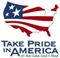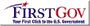Scientific Investigations Report 2006-5188

U.S. GEOLOGICAL SURVEY
Scientific Investigations Report 2006-5188

# Method For Estimating Trace-Metal Loads

Annual loads of dissolved and total cadmium (DCd and TCd), dissolved and total lead (DPb and TPb) and dissolved and total zinc (DZn and TZn) were estimated using the USGS software LOADEST, which uses instantaneous streamflow data and constituent concentrations to calibrate a regression model that describes constituent loads in terms of various functions of streamflow and time (Runkel and others, 2004). The software then uses the regression model to estimate loads over a user-specified interval for which daily mean streamflow data are provided. Model output includes statistical data to enable the user to evaluate the quality of the model. Model output also includes upper and lower 95‑percent confidence interval (CI) for the entire estimation period to provide an understanding of the precision of the estimates. In this study, separate regression models were calibrated for each constituent for each of the 10 sampling sites. Daily loads were calculated and summed to obtain annual loads.

The software performs calibration procedures and makes load estimates using four statistical estimation methods: Adjusted Maximum Likelihood Estimation (AMLE), Maximum Likelihood Estimation (MLE), Linear Attribution Method (LAM), and Least Absolute Deviation (LAD). The user chooses the most appropriate method for the data being analyzed. AMLE and MLE are suitable when the model calibration errors (residuals) are normally distributed; AMLE is the more appropriate method of the two when the calibration data set contains censored data (for example, data that are reported as below or above some threshold). LAM and LAD are useful when the residuals are not normally distributed. The AMLE estimation method was selected because in many cases the calibration data sets included censored data. The initial model calibration residuals for each constituent were tested for normality by plotting the natural log of the residuals for the AMLE model against their Z-scores, both given in the LOADEST output file. These plots yielded generally straight lines, indicating that the residuals were normally distributed.

LOADEST software allows the user to choose between selecting the general form of the regression from among several predefined models and letting the software automatically choose the best model, based on the Akaike Information Criterion (Akaike, 1981). The selection criterion is designed to achieve a good balance between using as many predictor variables as possible to explain the variance in load while minimizing the standard error of the resulting estimates. For this study, the software was allowed to choose the best model.

Output regression equations take the following general form:

ln(L) = a + b(ln Q) + c(ln Q2) + d[sin(2•T)] + e[cos(2•T)] + fT + gT2 (1)

where

 L is constituent load, in kg/d; Q is streamflow, in ft3/s; T is time in decimal years from beginning of calibration period; and a, b, c, d, e, f, and g are regression coefficients.

Some regression equations in this study did not include all of the above terms, depending on the particular model chosen by the software. A complete discussion of the theory and principles behind calibration and estimation methods used by the LOADEST software is given by Runkel and others (2004).U.S. Department of the Interior | U.S. Geological Survey